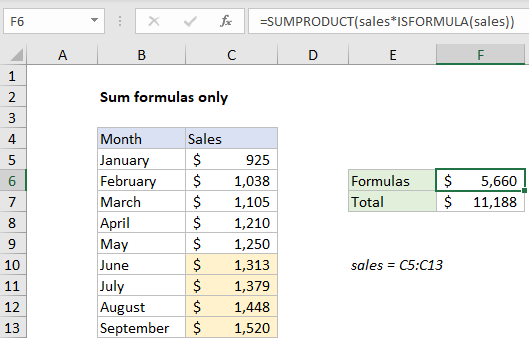## Excel Office

Excel How Tos, Tutorials, Tips & Tricks, Shortcuts

# Sum formulas only in Excel

This tutorial shows how to Sum formulas only in Excel using the example below;

## Formula

`=SUMPRODUCT(range*ISFORMULA(range))`## Explanation

To sum results from formulas only, you can use the SUMPRODUCT and ISFORMULA functions. In the example shown, the formula in F6 is:

`=SUMPRODUCT(sales*ISFORMULA(sales))`

where “sales” is the named range C5:C13, values in C5:C9 are hardcoded, and values in C10:C13 are generated with formulas.

Worked Example:   Cash denomination calculator in Excel

### How this formula works

This formula uses boolean logic to “filter” the numbers in column C before summing with the SUMPRODUCT function. When the formula is evaluated, values in the named range “sales” are expanded into an array:

`{925;1038;1105;1210;1250;1313;1379;1448;1520}`

To filter out any values that aren’t created by a formula, the ISFORMULA function is to create an array of TRUE FALSE values like this:

```=ISFORMULA(sales)
={FALSE;FALSE;FALSE;FALSE;FALSE;TRUE;TRUE;TRUE;TRUE}```

When these two arrays are multiplied by each other, the math operation coerces the TRUE and FALSE values to 1’s and 0’s:

```=SUMPRODUCT({925;1038;1105;1210;1250;1313;1379;1448;1520}*{0;0;0;0;0;1;1;1;1})
=SUMPRODUCT({0;0;0;0;0;1313;1379;1448;1520})```

The zeros cancel out non-formula results, and SUMPRODUCT sums and returns a final result.

Worked Example:   Sum if cells contain either x or y in Excel

### Not formulas

To sum values not generated by a formula, you can add the NOT function like this:

`=SUMPRODUCT(sales*NOT(ISFORMULA(sales)))`

Here, the NOT function reverses the TRUE FALSE results returned by ISFORMULA function, which filters out any values generated with a formula.